# How Much Solar Power Do I Need to Run a Refrigerator?

Many factors define how much solar power a refrigerator needs to run, from size and power rating to refrigeration system and duration of the operation. So when people ask, “how much solar power do I need to run a refrigerator” we answer them by asking specific questions about their refrigerator.

Once you know how much power your refrigerator consumes on average and set a daily operation duration, you can work out the projected energy consumption. With the projected energy consumption of the refrigerator, you know how much energy to expect from your solar panels. This will then guide you in calculating how many panels you need.

In determining how many solar panels you need, you must consider the power output of a single solar panel. It would help if you also considered the average daily duration of sunshine in your area. The product of the values of those two factors determines how much energy a single panel can produce. Knowing how much energy one solar panel can produce, you may then calculate how many solar panels you need to generate the total energy for the refrigerator.

## Calculating the Energy Requirement of Your Refrigerator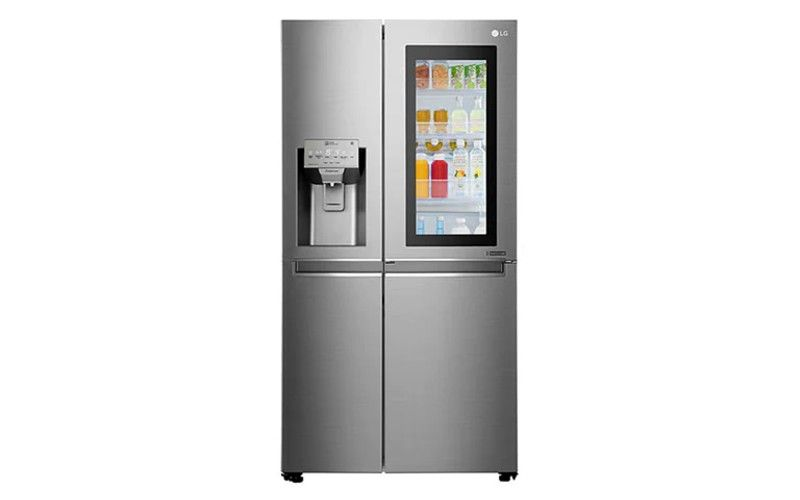In many cases, the energy rating of your refrigerator is boldly written on the energy rating sticker. This sticker is usually pasted on the refrigerator door. If yours happens to come with one, you will not have to do too many calculations.

The rating on the energy rating label is typically provided in kWh per year. You can determine the running watts (also called rated watts) from their value. Once you get the running watts, you may then multiply it by the number of hours you want the refrigerator to run per day. The product of this multiplication will give you the daily energy requirement of the refrigerator.

For example, you have one of the best energy-efficient solar refrigerators with an energy rating of 400 kWh per year.

To get its energy consumption per day, divide 400 by 365 days = 1.1 kWh per day.

Then to get its rated watts, divide 1.1 kWh by 24 hours = 0.046 kW.

Converted to watts, 0.046 kW equals 46 watts – this is the running watts of your refrigerator.

So, if you want your refrigerator to run for 10 hours a day, its daily energy needs would be:

46 x 10 =460 Wh or 0.46 kWh

If your refrigerator does not come with the sticker mentioned above, check the user’s manual for the energy rating. Alternatively, you may check for the quoted power rating written beside the serial number of the product.

If you still do not find the energy rating with the above, check for the voltage and current ratings of the refrigerator. By multiplying the values of these ratings, you can get the rated watts of the product.

For example, if the voltage rating of your refrigerator is 12 volts and the current rating is 3.83 amps, the rated watts would be:

12 x 3.83 = 46 W or 0.046 kW

If you want this refrigerator to run for 10 hours a day, its daily energy needs would be:

46 x 10 = 460 Wh or 0.46 kWh

## Different Types of Refrigerators and Their Power Needs

###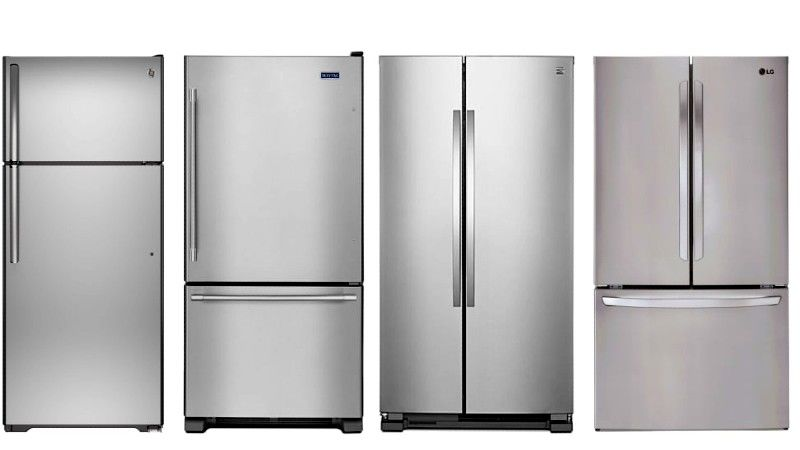How Voltage Rating Affects the Power Needs of Refrigerators

Refrigerators come with different voltage ratings, and these ratings define their power consumption. Generally, the higher the voltage rating, the more solar power you will need to run the refrigerator. Some of the common refrigerator ratings are 12 v, 24 v, 12/24 v, 48 v, 12/120 v, 110-120 v, and 220-240 v.

### How the Type of Current Affects the Power Needs of Refrigerators

Besides the voltage rating, some refrigerators, like RV refrigerators, run on direct current (DC). Their voltage ratings typically fall between 12 volts and 48 volts, and RV solar panels can power them in place of camping generators. These fridges can be powered directly by a solar battery or the current from a solar panel, so they do not necessarily need inverters.

On the other hand, AC refrigerators need an inverter to convert the DC solar power from the panels to AC before they can function. Their voltage ratings are usually 110-120 volts or 220-240 volts.

Then there are AC/DC refrigerators such as the 12/120v fridges. These types can run on both AC and DC. So, you may choose to provide power for both options, or you may choose one over the other.

### How the Refrigeration System Affects the Power Needs of Refrigerators

While most types of refrigerators run on electricity, some run on gas. In many cases, gas refrigerators are used in RVs. These RV refrigerators can come with the option to run on gas and electricity or gas only.

Those that run on gas and electricity typically use the direct current from a 12-volt battery when running on electricity. But the batteries usually die out faster because the absorption refrigeration system is more demanding.

### How the Location Affects the Power Needs of Refrigerators

Apart from the rating and features of refrigerators, ambient temperatures also affect how much power they need. Cooling in refrigerators is achieved by pumping heat out. But as the air surrounding the compressor becomes hotter, the refrigerator has to do more work to become cool. Consequently, it would need more power to run.

On the other hand, when the ambient temperature falls below a certain range (usually about 60 F), the compressor of combination refrigerators slows down. In such situations, the compressor will not run long enough to keep the refrigerator cool. Plus, the compressor may stop working at even lower temperatures as the temperature sensor becomes okay with the ambient temperature. If the compressor stops, the freezer compartment of the combination refrigerator will defrost, and power consumption will fall. Of course, without frost, it would not be a freezer.

So, when trying to run a refrigerator with solar power, you should consider the temperature where you will station it. The optimal ambient temperature for refrigerators is generally 60-95 degrees Fahrenheit. However, some manufacturers may specify the optimal range for their product in the users’ manual.

Apart from ambient temperature, the volume of ventilation affects the function of a compressor. Generally, compressors need a lot of ambient air to cool the refrigerator efficiently. So, when the refrigerator is boxed into a poorly ventilated corner, cooling efficiency drops. Consequently, the compressor has to do more work to pump air and keep the refrigerator cool, and more work equals increased power consumption.

## How To Set Up a Solar Energy System for Your Refrigerator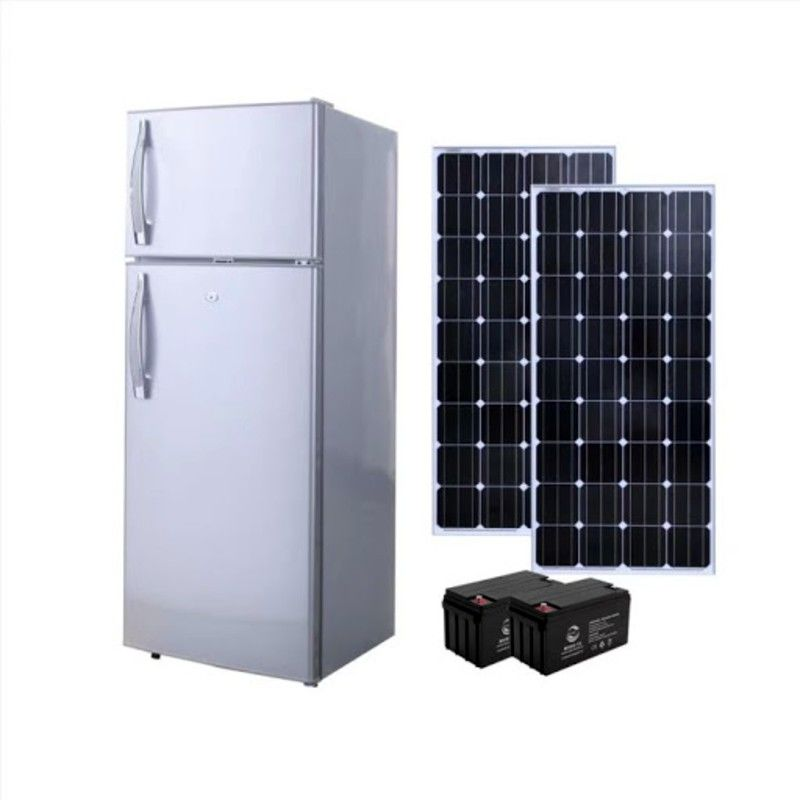You cannot run a refrigerator on solar panels alone. This holds because when the sun goes down, there will be no solar energy for the panels to power the refrigerator. To this end, you must set up a solar system, including solar batteries, an inverter, and a charge controller for your refrigerator.

The solar panels produce solar power in such a system, and the battery bank stores them as electrical energy. So, even when there is no sun, the battery will power the refrigerator.

Some solar refrigerators solve the problem highlighted in the paragraphs above. They come with their own solar panels and battery, and they are typically used outdoors. So, you do not have to set up a solar system for them.

### Inverter

#### What Does The Inverter Do?

The inverter’s role in the solar power system is to convert the DC power coming from the battery to AC power for your refrigerator. So, if you are using an AC refrigerator, you will need an inverter because the direct current (DC) from the battery and solar panel cannot power an AC refrigerator. However, if your refrigerator runs on direct current, you do not need an inverter.

In converting DC to AC, there will be some power loss. Excessive power loss will markedly reduce the efficiency of your solar panel system. But you can mitigate this by getting a highly efficient inverter.

#### Size/Rating of the Inverter

Apart from efficiency, the size of the inverter also matters. The size of the inverter you get depends on the running voltage of the battery and the maximum starting watts of the refrigerator.

Generally, the inverter and the battery should have the same voltage. However, you may overestimate the voltage of the inverter in case you want to use other devices on the solar power setup.

Refrigerators have maximum starting watts, also called surge watts. The surge watts are the power needed by a refrigerator to start, and their value is always higher than the rated watts.

The maximum load of the inverter you get should be enough to handle the maximum starting watts of your refrigerator. This is particularly important if you will be using other devices on the solar energy system.

You can estimate the surge watts of your refrigerator by multiplying by the value of the rated watts by 1.5. So, in the 46-watt refrigerator above, the surge watts would be 46 x 1.5 = 69 watts. For this refrigerator, the maximum load of the inverter should be at least 2 times the surge watts. So, a 150-watt inverter would do.

The bat

### Battery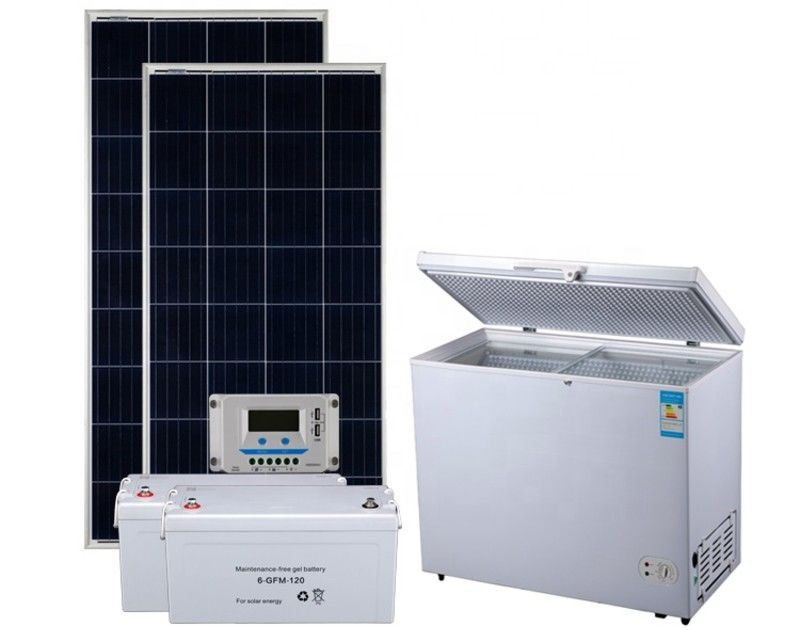The battery will store energy from the solar panels and supply power to the refrigerator when there is no sun. However, the output of the system will depend on the capacity of the battery.

#### What Is the Right Battery Capacity for My Refrigerator?

Before you can choose the battery capacity for your refrigerator, you must know the daily needs of the refrigerator. This section will use the daily energy consumption calculated for the refrigerator in the example above (0.46 kWh or 460 Wh).

Batteries come with various voltage ratings: 12 volts, 24 volts, 48 volts, etc. In most cases, solar batteries are rated 12 volts. So, you may opt for that.

However, it does not end there. You must calculate the battery capacity that will suit your refrigerator. The battery capacity is typically provided in Ah, and to calculate how much of it you need, use the following formula:

Daily energy consumption of the refrigerator ÷ voltage of the solar battery

460/12 = 38.3 Ah

For the refrigerator in the example, you need a 12v battery with a capacity of at least 38.3 Ah. So, a 12v 50Ah battery will do.

To lengthen the lifespan of a battery, we recommend you get one with a capacity twice the daily energy required by the refrigerator. So, for our refrigerator, a 12v 100Ah battery is the best option.

### Charge Controller

When setting up a solar system to run a refrigerator, you need a charge controller. As the name says, charge controllers control the flow of the solar power getting to batteries and ensure they do not get overloaded.

Charge controllers come in different voltage ratings, including 12 volts, 24 volts, and 36 volts. Opt for one that has the same voltage rating as the inverter and battery.

Apart from that, the current capacity of your charge controller should be able to handle the short circuit current (ISC) of the solar panels. Generally, the current capacity of the charge controller should be at least 1-1.25 the ISC of the solar panels.

### Solar Panels

#### How Much Solar Power Do I Need to Run My Refrigerator?

So, we will run through this section using the value for the daily energy needs from the examples above (0.46 kWh or 460 Wh).

To calculate how many solar panels you need, you have to determine the solar power output of each one of the panels you have. Once you know how much power each solar panel produces and the average daily duration of sunshine, you can determine how many solar panels you need for your refrigerator.

So, say you have a 30-watt solar panel, and the sun shines for an average of 4 hours daily. The number of solar panels you will need for the 0.46 kWh (460 Wh) in the example above will be as follows:

The daily energy requirement of the refrigerator (Wh) ÷ power output/rating of a single solar panel (W) ÷ average daily duration of sunshine (h)

460 ÷ 30 ÷ 4 = 3.83.

For the refrigerator in the example above, you need at least four 100-watt solar panels (3.83 is approximated to 4) to run the refrigerator. You may get more solar panels if you intend to power other devices with the solar power system.

The average refrigerator can be powered by 3 to 4 average solar panels. This estimation is based on a refrigerator that needs 3.8-kWh per day and solar panels that produce up to 1-kWh per day. Since this average refrigerator needs 3.8 kWh per day and one solar panel provides 1 kWh per day, one will need 3.8 ÷ 1 (3.8) solar panels to run a refrigerator with such an energy rating.

## How Long Will a 12 Volt Battery Run a Refrigerator?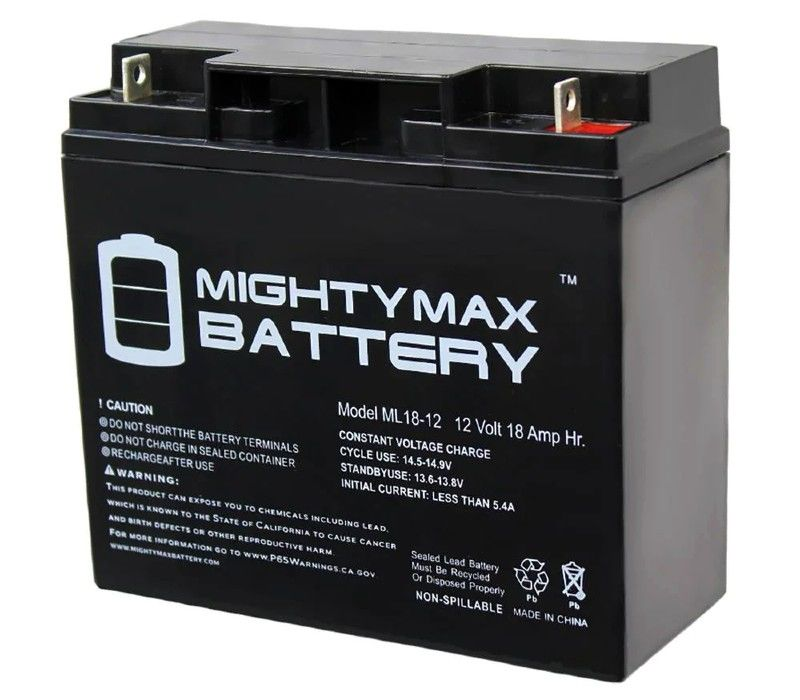A 12-volt car battery typically has an amperage of 48 Ah. Such a battery can produce up to 576 Wh (48 x 12) when fully charged.

So, say you have a refrigerator that has a running watt of 46 watts. To calculate how long the 12v 48Ah battery will power it, divide the full-charge energy of the battery by the running watts of the refrigerator.

In this case, the battery will run the refrigerator for 576 ÷ 46 = 12.5 hours. So, the 12v battery will run the refrigerator for up to 12 hours when fully charged.

## What Size Solar Panel Do I Need to Run a 12v Fridge?

The size of the solar panel you need to run a 12v fridge depends on the daily energy needs of the fridge. It also depends on the average daily duration of sunshine in your region.

So, we know the fridge is 12v, but we do not know its wattage or amperage. So, we will assume the amperage is 4 amps. This will make the wattage of the 12v fridge = 12 x 4 = 48 watts.

Now, if you let this 12v fridge run for 6 hours a day, its daily energy consumption would be 48 x 6 = 288 Wh.

Then if the average daily duration of sunshine in your area is 8 hours, the size of the solar panel you need for the fridge would be:

288 ÷ 8 = 36 watts.

To run a refrigerator rated 12v, 4 amps for 6 hours a day with daily sunshine of 8 hours, you need a 36-watt solar panel.

## Final Thoughts

Determining how much solar power or how many solar panels you need to run your refrigerator is a stepwise but simple process. The first step is to determine the daily energy consumption; you can calculate this following the steps we outlined above. Once you know how much energy the refrigerator needs daily, you know how much solar power you need to run it. Then, you can now calculate how many panels you need to run it.